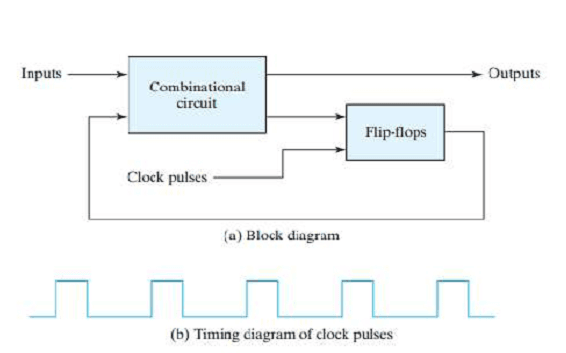# Sequential Logic Circuits A+ A  A-

Till now we studied the logic circuits whose outputs at any instant of time depend only on the input signals present at that time are known as combinational circuits. Moreover, in a combinational circuit, the output appears immediately for a change in input, except for the propagation delay through circuit gates.

On the other hand, the logic circuits whose outputs at any instant of time depend on the present inputs as well as on the past outputs are called sequential circuits. In sequential circuits, the output signals are fed back to the input side. A block diagram of a sequential circuit is shown in Figure below:-It consists of a combinational circuit to which storage elements are connected to form a feedback path. The storage elements are devices capable of storing binary information.

The binary information stored in these elements at any given time defines the state of the sequential circuit at that time. The sequential circuit receives binary information from external inputs that, together with the present state of the storage elements, determine the binary value of the outputs.

These external inputs also determine the condition for changing the state in the storage elements. The block diagram demonstrates that the outputs in a sequential circuit are a function not only of the inputs, but also of the present state of the storage elements. The next state of the storage elements is also a function of external inputs and the present state. Thus, a sequential circuit is specified by a time sequence of inputs, outputs, and internal states.

There are two types of sequential circuits, and their classification is a function of the timing of their signals.

Asynchronous sequential circuit:

A sequential circuit whose behavior depends upon the sequence in which the input signals change is referred to
as an asynchronous sequential circuit. The output will be affected whenever the input changes. The commonly
used memory elements in these circuits are time-delay devices.

There is no need to wait for a clock pulse. Therefore, in general, asynchronous circuits are faster than synchronous sequential circuits. However, in an asynchronous circuit, events are allowed to occur without any synchronization. And in such a case, the system becomes unstable. Since the designs of asynchronous circuits are more tedious and difficult, their uses are rather limited. The memory elements used in sequential circuits are flip-flops which are capable of storing binary information.

Synchronous sequential circuit:

A sequential circuit whose behavior can be defined from the knowledge of its signal at discrete instants of time is referred to as a synchronous sequential circuit.

In these systems, the memory elements are affected only at discrete instants of time. The synchronization is achieved by a timing device known as a system clock, which generates a periodic train of lock pulses. The outputs are affected only with the application of a clock pulse. The storage elements (memory) used in clocked sequential circuits are called flip flops